## Sunday, December 30, 2007

### Roots of Unity

For n ≥ 3, xn = 1 has three or more distinct roots. While (1, -1) were well known during Abraham de Moivre's day. The other roots were not so well known. It took de Moivre's formula to establish these roots on a firm footing. Roger Cotes had independently discovered the properties of the roots of unity as demonstrated by his formulas. Unfortunately, he had died before he could explain many of his discoveries in detail. It was left to de Moivre to explain Cotes' formulas.

In a previous blog, I showed how de Moivre came up with his famous formula based on the work of Francois Viete. In today's blog, I will show how he provided a proof for the existence of the roots of unity.

In 1739, de Moivre used his formula to find the nth root for any complex number (a + bi).

Theorem 1: For any complex number, there are n distinct nth roots.

if a,b ≠ 0, then:where:

0 ≤ k ≤ n-1

Proof:

(1) Let a,b be real numbers such that either a ≠ 0 or b ≠ 0

(2) We note first that:(3) Since: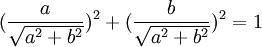It is clear that:And further that: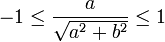(4) Therefore, there exists φ such that [see here for review of cosine if needed]:(5) Since cos2(φ) + sin2(φ) = 1 (see Corollary 2, here), we can see that:

sin2(φ) = 1 - cos2(φ) =(6) So that we also have now: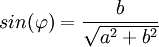(7) We can now conclude: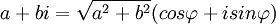since: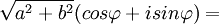(8) Using De Moivre's formula (see Theorem 1, here), we know that:(9) From the basic properties of sin and cosine, we know that:

cos(φ + 2kπ) + isin(φ 2kπ) = cos(φ) + isin(φ)

(10) Multiplying a2 + b2 to both sides gives us:(11) Combining step #7 with step #10 gives us:(12) Now, we know that each of the values are distinct for 0 ≤ k ≤ n-1 since none of the angles vary by a multiple of .

QED

Corollary 1.1: Roots of Unity

There are n roots of unity such that: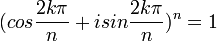where:

0 ≤ k ≤ n-1

Proof:

(1) Let a=1 and b=0

(2) Then a+bi = 1+0*i = 1

(3) Using Theorem 1 above, we have:(4) Using step #4 and step #6 in Theorem 1 above, we have: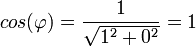and(5) So, φ = 0

(6) Since: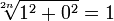we are left with:where:

0 ≤ k ≤ n-1

QED

Corollary 1.2: Roots of -1

There are n roots of unity such that: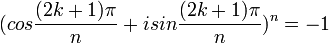where:

0 ≤ k ≤ n-1

Proof:

(1) Let a=-1 and b=0

(2) Then a+bi = -1+0*i = -1

(3) Using Theorem 1 above, we have:(4) Using step #4 and step #6 in Theorem 1 above, we have:and(5) So, φ = π

(6) Since: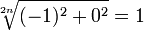we are left with:where:

0 ≤ k ≤ n-1

QED

References
• Jean-Pierre Tignol, Galois' Theory of Algebraic Equations, World Scientific, 2001

i want some more information about that book that is 1000 or more pages by pierre de fermat.
and some mroe about dr wise.

thanks

Larry Freeman said...

Pierre de Fermat only published one proof which I have already blogged about.

I assume that you are referring to the 100-page proof by Professor Andrew Wiles of Fermat's Last Theorem. Here is a link if you would like to go to it directly.

There are also excellent web pages that provide a good overview of Wiles' proof. Here's one to look at. And here's another. Hopefully, these links address your question. :-)

I am going through the history of group theory which is needed to understand Wile's very complex proof.

My goal is to provide a complete proof including all of the underlying mathematical assumptions.

I am still working through Kummer's proof which was the most important proof prior to Wiles' brilliant work.

Cheers,

-Larry